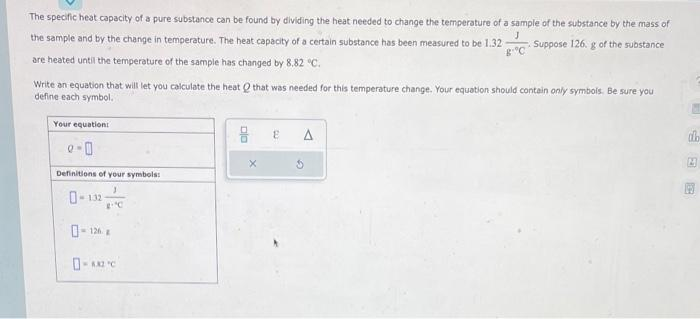Home / Expert Answers / Chemistry / the-specific-heat-capacity-of-a-pure-substance-can-be-found-by-dividing-the-heat-needed-to-change-t-pa999

# (Solved): The specific heat capacity of a pure substance can be found by dividing the heat needed to change t ...The specific heat capacity of a pure substance can be found by dividing the heat needed to change the temperature of a sample of the substance by the mass of the sample and by the change in temperature. The heat capacity of a certain substance has been measured to be $$1.32 \frac{\mathrm{J}}{\mathrm{g}={ }^{\circ} \mathrm{C}}$$, Suppose $$126 . g$$ of the substance are heated until the temperature of the sample has changed by $$8.82^{\circ} \mathrm{C}$$. Write an equation that will let you calculate the heat $$Q$$ that was needed for this temperature change. Your equation should contein only symbois. Be sure you define each symbol.

We have an Answer from Expert